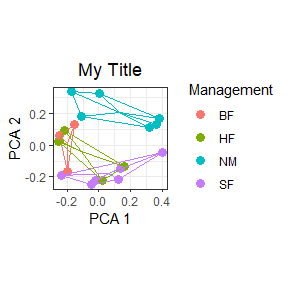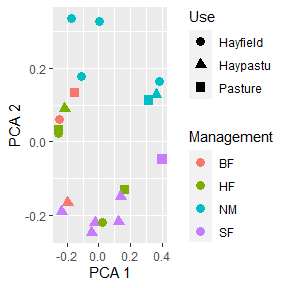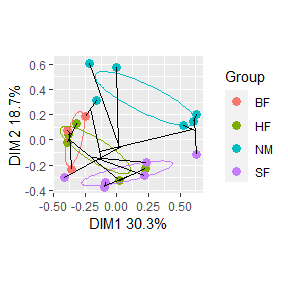# Introduction

To enable further customization, all functions in this package return silently a list of the data frames used for the plots and the plots themselves These may be captured by assigning the function result to a variable. For gg_ordiplot, for example, get a list of the names of the items returned:

suppressPackageStartupMessages(library(vegan))
suppressPackageStartupMessages(library(ggplot2))
suppressPackageStartupMessages(library(ggordiplots))
data("dune")
data("dune.env")
dune.hel <- decostand(dune, method = "hellinger")
ord <- rda(dune.hel)
my.plot <- gg_ordiplot(ord, groups = dune.env$Management, hull = TRUE, spiders = TRUE, ellipse = FALSE, plot = FALSE) names(my.plot) ##  "df_ord" "df_mean.ord" "df_ellipse" "df_hull" "df_spiders" ##  "plot" The last object in the list is the plot itself. You can capture the plot and make modifications by adding ggplot2 labels and themes: a.plot <- my.plot$plot
a.plot + theme_bw() + labs(color = "Management", x = "PCA 1", y = "PCA 2", title = "My Title") +
theme(plot.title = element_text(hjust = 0.5))  # centers main title, ggplot2 version 2.2+The other items in the list are the data frames used for the plot layers:

Item Description
df_ord Point coordinates for ordination with Group variable
df_mean.ord Label coordinates
df_ellipse Data for plotting ellipses
df_hull Data for plotting hulls
df_spiders Data for plotting spiders

You can check the first few lines of each data frame thus:

head(my.plot$df_spiders) ## Group cntr.x cntr.y x y ## 2 BF -0.1995202 0.009619176 -0.1970059 -0.16455540 ## 10 BF -0.1995202 0.009619176 -0.2505024 0.06096196 ## 11 BF -0.1995202 0.009619176 -0.1510522 0.13245097 ## 5 HF -0.1070437 -0.041316511 -0.2532165 0.02333147 ## 6 HF -0.1070437 -0.041316511 -0.2152557 0.09089893 ## 7 HF -0.1070437 -0.041316511 -0.2497939 0.03067965 The ggplot2 statements I used for each layer are: # Basic ordination plot: xlab <- paste("Axis", choices, sep = " ") ylab <- paste("Axis", choices, sep = " ") geom_point(data = df_ord, aes(x = x, y = y, color = Group), size = 3) + xlab(xlab) + ylab(ylab) # Plot ellipses: geom_path(data = df_ellipse, aes(x = x, y = y, color = Group), show.legend = FALSE) # Plot centroid labels: geom_text(data = df_mean.ord, aes(x = x, y = y, label = Group, color = Group), show.legend = FALSE) # Plot hulls: geom_path(data = df_hull, aes(x = x, y = y, color = Group), show.legend = FALSE) # Plot spiders: geom_segment(data = df_spiders, aes(x = cntr.x, xend = x, y = cntr.y, yend = y, color = Group), show.legend = FALSE) # Plot cluster segments: geom_segment(data = df_segments, aes(x = x, y = y, xend = xend, yend = yend)) With the above information and some knowledge of ggplot2 you can modify the plots however you wish. And to learn more, you can inspect the code by entering gg_ordiplot or any other function written in R (i.e. not compiled) without parentheses. As a short example, the hidden function in vegan for generating data for an ellipse is: vegan:::veganCovEllipse ## function (cov, center = c(0, 0), scale = 1, npoints = 100) ## { ## theta <- (0:npoints) * 2 * pi/npoints ## Circle <- cbind(cos(theta), sin(theta)) ## Q <- chol(cov, pivot = TRUE) ## o <- attr(Q, "pivot") ## t(center + scale * t(Circle %*% Q[, o])) ## } ## <bytecode: 0x000000003f8ad980> ## <environment: namespace:vegan> # Examples Say you want to make an ordination plot with points distinguished by two variables assigned to symbol shape and symbol color. You can do this by extracting df_ord from a plot result and adding a second grouping variable. Continuing with the example from above: ord.data <- my.plot$df_ord
head(ord.data)
##             x           y Group
## 1 -0.23409791 -0.19110003    SF
## 2 -0.19700590 -0.16455540    BF
## 3 -0.04472727 -0.24679380    SF
## 4 -0.02267027 -0.22032168    SF
## 5 -0.25321647  0.02333147    HF
## 6 -0.21525573  0.09089893    HF
ord.data$Use <- dune.env$Use
colnames(ord.data) <- c("x", "y", "Management", "Use")
head(ord.data)
##             x           y Management      Use
## 1 -0.23409791 -0.19110003         SF Haypastu
## 2 -0.19700590 -0.16455540         BF Haypastu
## 3 -0.04472727 -0.24679380         SF Haypastu
## 4 -0.02267027 -0.22032168         SF Haypastu
## 5 -0.25321647  0.02333147         HF Hayfield
## 6 -0.21525573  0.09089893         HF Haypastu
ggplot(data = ord.data, aes(x = x, y = y, color = Management, shape = Use)) + geom_point(size = 3) +
xlab("PCA 1") + ylab("PCA 2")
## Warning: Using shapes for an ordinal variable is not advisedAs long as you start from the same data, you can take plots and data frames generated with different functions and combine them in new ways. For example, add a dendrogram to an ellipse plot.

data("dune")
data("dune.env")
dune.bray <- vegdist(dune, method = "bray")
ord <- cmdscale(dune.bray, k = nrow(dune) - 1, eig = TRUE, add = TRUE)
cl <- hclust(dune.bray, method = "single")
clstr.plot <- gg_ordicluster(ord, cluster = cl, plot = FALSE)
ellipse.plot <- gg_ordiplot(ord, groups = dune.env$Management, plot = FALSE) ellipse.plot$plot + geom_segment(data = clstr.plot\$df_segments, aes(x = x, y = y,
xend = xend, yend = yend))Enjoy!